Home

# Sur le comportement, par torsion, des facteurs epsilon de paires

## Résumé

Soient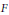$F$ un corps commutatif localement compact non archimédien et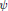$\psi$ un caractère additif non trivial de$F$ . Soient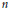$n$ et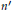${n}'$ deux entiers distincts, supérieurs à 1. Soient$\pi$ et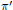${\pi }'$ des représentations irréductibles supercuspidales de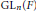$\text{G}{{\text{L}}_{n}}\left( F \right)$ ,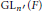$\text{G}{{\text{L}}_{{{n}'}}}\left( F \right)$ respectivement. Nous prouvons qu’il existe un élément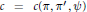$c=c\left( \pi ,{\pi }',\psi \right)$ de${{F}^{\times }}$ tel que pour tout quasicaractère modéré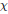$\mathcal{X}$ de${{F}^{\times }}$ on ait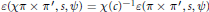$\mathcal{E}\left( \chi \pi \times {\pi }',s,\psi \right)=\chi {{\left( c \right)}^{-1}}\mathcal{E}\left( \pi \times {\pi }',s,\psi \right)$ . Nous examinons aussi certains cas où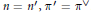$n={n}',{\pi }'={{\pi }^{\text{v}}}$ . Les résultats obtenus forment une étape vers une démonstration de la conjecture de Langlands pour$F$ , qui ne fasse pas appel à la géométrie des variétés modulaires, de Shimura ou de Drinfeld.

Abstract

Let$F$ be a non-Archimedean local field, and$\psi$ a non-trivial additive character of$F$ . Let$n$ and${n}'$ be distinct positive integers. Let$\pi$ ,${\pi }'$ be irreducible supercuspidal representations of$\text{G}{{\text{L}}_{n}}\left( F \right)$ ,$\text{G}{{\text{L}}_{{{n}'}}}\left( F \right)$ respectively. We prove that there is$c=c\left( \pi ,{\pi }',\psi \right)$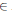$\in$${{F}^{\times }}$ such that for every tame quasicharacter$\mathcal{X}$ of${{F}^{\times }}$ we have$\mathcal{E}\left( \chi \pi \times {\pi }',s,\psi \right)=\chi {{\left( c \right)}^{-1}}\mathcal{E}\left( \pi \times {\pi }',s,\psi \right)$ . We also treat some cases where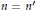$n={n}'$ and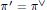${\pi }'={{\pi }^{\text{V}}}$ . These results are steps towards a proof of the Langlands conjecture for$F$ , which would not use the geometry of modular—Shimura or Drinfeld—varieties.

• # Send article to Kindle

Note you can select to send to either the @free.kindle.com or @kindle.com variations. ‘@free.kindle.com’ emails are free but can only be sent to your device when it is connected to wi-fi. ‘@kindle.com’ emails can be delivered even when you are not connected to wi-fi, but note that service fees apply.

Find out more about the Kindle Personal Document Service.

Sur le comportement, par torsion, des facteurs epsilon de paires
Available formats
×

# Send article to Dropbox

To send this article to your Dropbox account, please select one or more formats and confirm that you agree to abide by our usage policies. If this is the first time you use this feature, you will be asked to authorise Cambridge Core to connect with your <service> account. Find out more about sending content to Dropbox.

Sur le comportement, par torsion, des facteurs epsilon de paires
Available formats
×

# Send article to Google Drive

To send this article to your Google Drive account, please select one or more formats and confirm that you agree to abide by our usage policies. If this is the first time you use this feature, you will be asked to authorise Cambridge Core to connect with your <service> account. Find out more about sending content to Google Drive.

Sur le comportement, par torsion, des facteurs epsilon de paires
Available formats
×

## References

Hide All
MathJax
MathJax is a JavaScript display engine for mathematics. For more information see http://www.mathjax.org.

# Sur le comportement, par torsion, des facteurs epsilon de paires

## Metrics

### Full text viewsFull text views reflects the number of PDF downloads, PDFs sent to Google Drive, Dropbox and Kindle and HTML full text views.

Total number of HTML views: 0
Total number of PDF views: 0 *Loading metrics...

### Abstract viewsAbstract views reflect the number of visits to the article landing page.

Total abstract views: 0 *Loading metrics...

* Views captured on Cambridge Core between <date>. This data will be updated every 24 hours.

Usage data cannot currently be displayed